In Lean Six Sigma a process capability always explains the capability of the process performing at one particular point in time.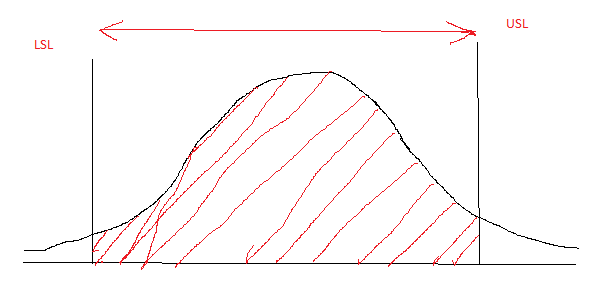Figure 1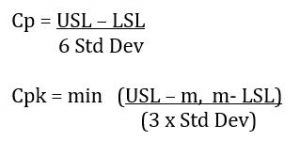A process capability is always calculated considering the points or data which falls within the specification limit, explicating how much of the process is capably producing with respect to the customer’s specification. In other words, process capability takes into consideration the  that are able to be achieved.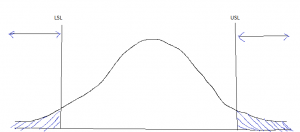Figure 2

Sigma level on the other hand takes into consideration the probabilities of failures instead, subject to the current capability level.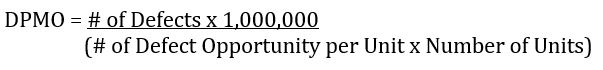It is necessary to estimate Z bench in order to obtain the failure rates at each end of the continuum;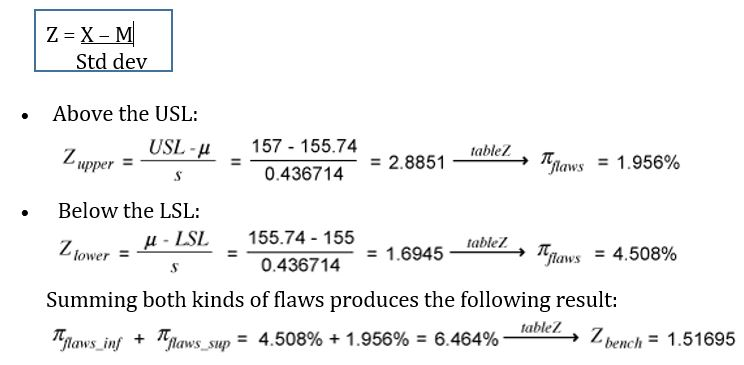Source: isixsigma.com webpage

Z- Table (source: http://www.z-table.com/)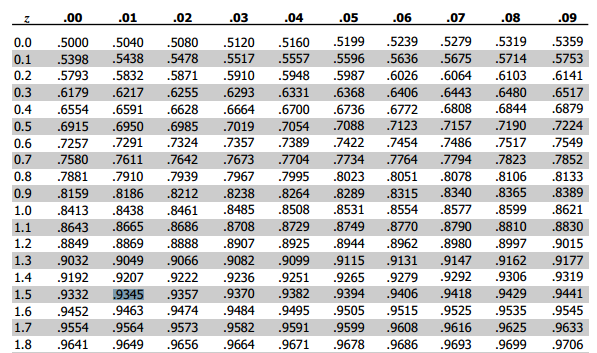Adding the 1.5 sigma shift the sigma level of the process will stand at 3.1695.

The difference between process capability and sigma level is synonymous to the case of a glass half full (figure 1) of water or one with half empty (figure 2). A half full glass measures the capability of the process with respect to the potential or how much is filled currently. Whereas the latter simply means how much more is missing from the potential. Sigma level gives an overall view of the process’ performance considering the defects amounted for at the present capability. Process capability gives us the idea of process performance being able to provide with respect to what is needed.

So if you’re considering the case of half glass of water, which is of greater significance? Being able to produce to the current capability is a realization. How much of the capability is not being met and the amount of defects shows how much more is yet to be improved. The defects resemble the target for improvement thus a greater importance shown as the overall competence of the process.

In summary:

Process Capability in Lean Six Sigma is the quantifiable comparison between the variations in the Process (Voice of the Process) to its allowed variation limits (Voice of Customer).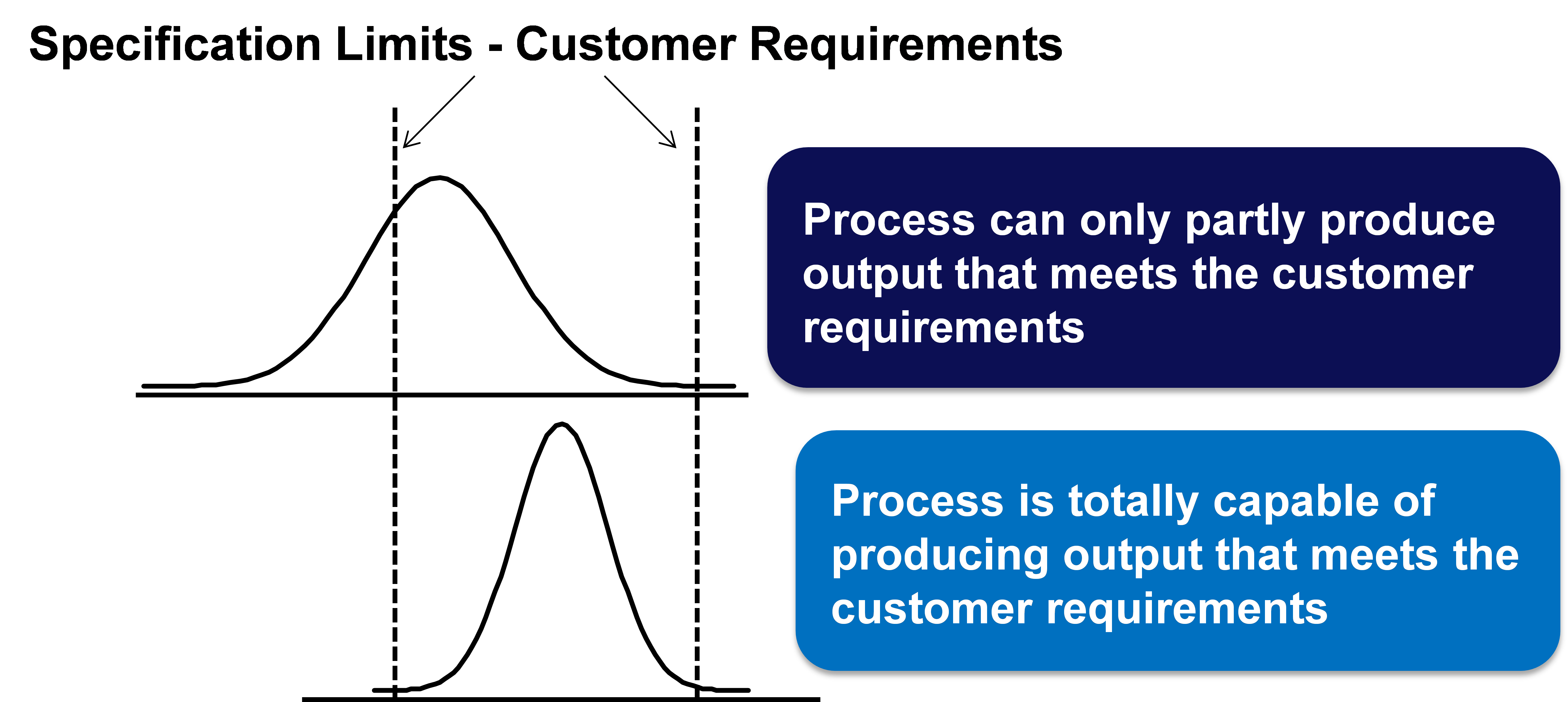Figure 3

Capability statistics estimate the probability that a process will be in specification.

Since the calculation uses probability distributions, there are two requirements for the statistic to be valid:

• The process must be in control
• The process must follow the distribution used in the calculation (usually the normal distribution)

By: Dr. Satnam Singh, PhD (Managing Director MBizM Group) & Muraliraj Jagantheran (M.Ec, ICBB) (PhD Candidate – PhD (Economics), University Malaya)